Theoretical Dialectical Journal: Physics-Mathematics-Logic-Philosophy

English-Russian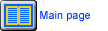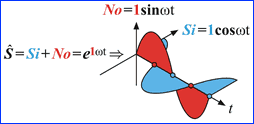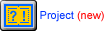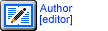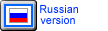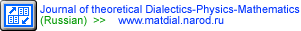Select issue Issue 1 Issue 2> Issue 3>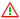Resume of issue In this journal the bases of the differentially integral logic and philosophy of physical processes, both continuous and discontinuous are considered. This system is named as the dialectic analysis, or dialectics, as the series of its axioms belongs to Hegel's dialectical phylosophy and logic. As a special case, Hegel's dialectics includes Aristotle's logic and metaphysics. Methods of dialectical analysis possess the broad conceptual and mathematical apparatus which contains both classical derivatives of continuous processes and discrete derivatives of discrete processes. The mathematical basis of dialectics is the quantitatively qualitative numerucal field, which includes, as a specific and very limited case, the field of complex numbers. The quantitatively qualitative numerical field is more genuine and obvious than the field of complex numbers. In the definite sense, the numerical field is analogous to the electromagnetic field. The numerical field of dialectics and its logical apparatus make it possible to describe and solve many problems in the simplest and most comprehensive way, such as-: physical, technical, technological and those which could not be solved by classical analysis. The field of quantitatively qualitative numbers allows us to consider two principal types of continuity and discreteness: additive and multiplicative. Therefore, this field considers both the classical differentials, derivatives, and integrals of continuous sums and the multiplicative differentials, derivatives, and integrals of continuous products. Although the multiplicative differentially integral calculus can be expressed by the classical additive differentially integral calculus, the two calculuses differ in principle. The multiplicative calculus allows us to see a great many facts which would be impossible to find by the classical additive calculus. The logical algebra of dialectics operates discontinuous, continuous and discontinuously continuous judgements, whereas the mathematical logic is based on two elementary constant judgements with measures 1 and 0. This means that dialectical analysis makes it possible in principle to develop new structures of microprocessors and effective methods of computer programming. They will allow us to adequately model (describe) intellectual processes first of all on the intuitive level where the logic of thinking is most effective. Note that the laws of the intuitive level of thinking are concerned with the level of the Universe. The fields of quantitatively qualitative differentially integral judgements and numbers are the "Physics" of logical thinking, i.e. the mathematical image of real logical processes. Therefore, without dialectical analysis, it is impossible to create an artificial intellect in the deep sense of the word. It is also impossible to make the essential theoretical and practical progress for understanding of the atomic and elementary particles structure where superhigh frequencies and vast speeds play a role. All subsequent articles are written in language of dialectics and a dialectic binary numerical wave field,and assume knowledge of articles published here.
Issue 1
MATHEMATICAL EXPRESSION OF THE MAIN CATEGORIES OF DIALECTICAL PHILOSOPHY AND LOGIC
Contents

full text>
HTML-format
PDF-format
1. Introduction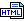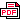2. Dialectical Judgements and the Quantitative-Qualitative Numerical Field3. Quantitative-qualitive relation and operations, connecting dialectic judgements and their measures4. The fundamental period of the World and ancient metrics5. Mathematical structure of elementary variable judgements of dialectical logic6. Continuous and discontinuous differentials and derivatives of judgements of dialectical logic7.1. Additive and multiplicative judgements of dialectical logic7.2. Additive and multiplicative differentials and integrals of dialectical judgements8. A Field of judgements of dialectical logic. Circulations and semi-circulations of dialectical judgements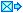kreidik@rambler.ru